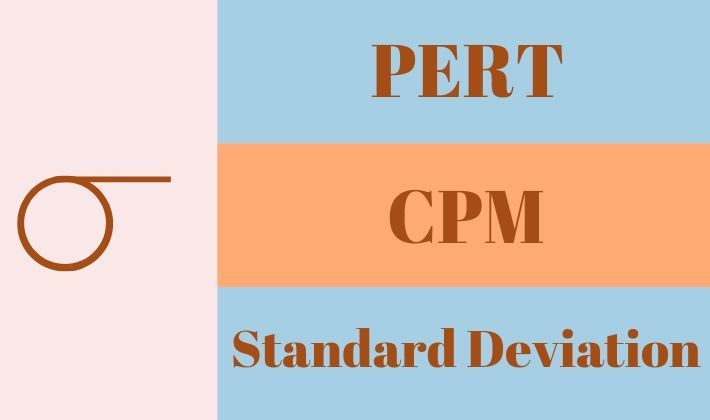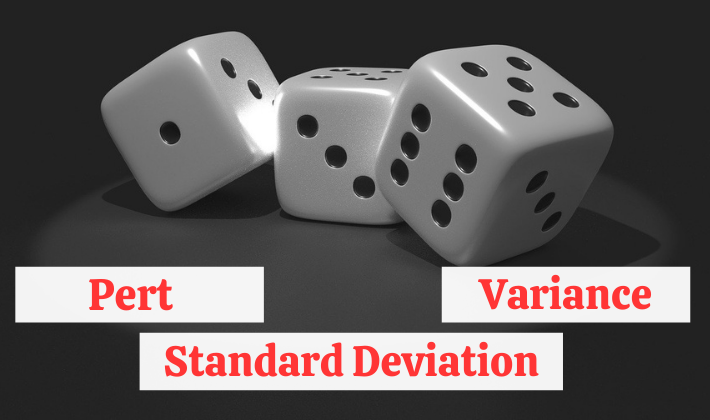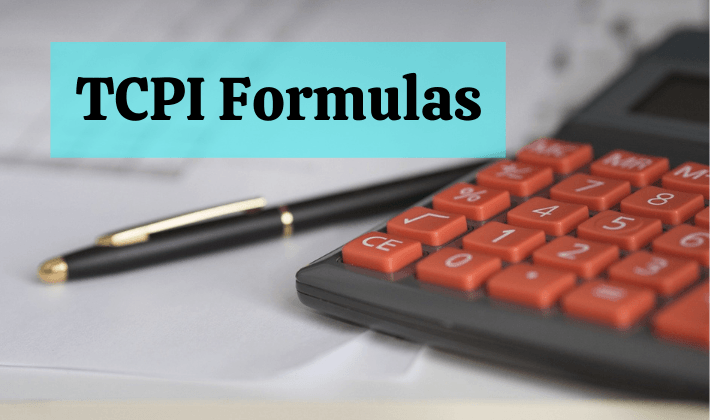## Earned Value Management Formulas

Quick Reference for Earned Value Management Formulas If you are looking for a comprehensive list of all the Earned Value Management Formulas, then you are at the right place. If you are preparing for PMP® Exam, you will find all the Earned Value Formulas. This article would provide you with a step up in doing…## Estimate to Complete (ETC) Formulas In Project Management

Estimate To Complete (ETC) is an estimation of funds required to complete the remaining work of a project. I have written this post to explain ETC with the help of an example. You will find a complete discourse on ETC in this post, which includes: significance of ETC in project management. definition and meaning of…## Three Point Estimate Formulas

Quick Reference on Three Point Estimate I have written a series of articles on Three Point Estimate and related concepts. Before reading this, you should look at my first article of the series. I have explained the basic concept of Three Point Estimate in my first article. I have also explained that PERT is not…## Earned Value Management System Explained in Easy Language

Do you find Earned Value Management System a difficult concept? I generally ask the above question in my class before I start explaining what is Earned Value Management System. Usually I find most of the students have not even heard about Earned Value Management System, let alone gauge its difficulty. On the other hand, a small…## How To Use Analogous & Parametric Estimating Techniques?

Project estimation is the most fundamental aspect of Project Management (PM). Analogous and parametric estimating techniques are most commonly used methods. You will find definitions, detailed description, and examples of these techniques in this post. You will also get to know about the difference between Analogous and parametric estimation. This post should help PMP aspirants,…## Using PERT & Standard Deviation For Critical Path Analysis

You would find Program Evaluation & Review Technique (PERT), Critical Path Method (CPM) and Standard Deviation in complete detail this post. IT describes how they are related to each other and how they can be used together for project schedule analysis. This article assumes that you are already know how to use PERT Formula for…## Variance And Standard Deviation In PERT Formula [PMP]

You might be looking to understand the usage of variance and standard deviation in PERT formula. PERT is an acronym for Program Evaluation and Review technique. This technique is used to determine duration and cost estimates of a task. In this technique, a weighted average (mean) of three different estimates is used to determine the…## How To Use PERT Formula For Three Point Estimating?

PERT Formula is one on the ways for doing three point estimation. It calculates expected duration by finding the weighted average of three different estimates viz. Optimistic (O), Pessimistic (P), and Most Likely (M). E_PERT=(O+P+4×M)/6 The three point estimation can also be done by using simple average formula. E_SA=(O+P+M)/3 PERT (Program Evaluation and Review Technique)…## To Complete Performance Index (TCPI) Formulas | PMP Exam

To Complete Performance Index (TCPI) is the estimated cost efficiency required to complete the remaining project work within a defined budget. The defined budget could be the original budget i.e., Budget At Completion (BAC) or it could be the revised budget Estimate At Completion (BAC). TCPI is different from Cost Performance Index (CPI). TCPI is…## Estimate At Completion (EAC) Formulas/Calculation For PMP

Estimate At Completion is the revised estimate of the total funds required to complete total work of a project. It is the sum of the Actual Cost (expenditure already incurred or the money already spent) till the control date and Estimate to Complete (expected cost of remaining work). EAC formula can be expressed by using…